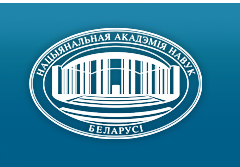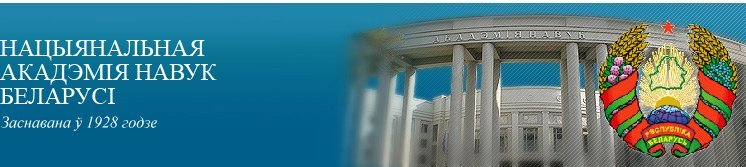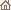Галоўная Навіны Аб акадэміі Дзейнасць акадэміі Члены акадэміі Арганізацыі Выданні акадэміі Мерапрыемствы Кантакты Электронныя звароты Даведачная інфармацыя Карта сайта

# Весці Нац. акадэміі навук Беларусі. Сер. фіз.-матэм. навук, 2000, No.2/ Выданні акадэміі / Навуковыя часопісы

Весці Нац. акадэміі навук Беларусі. Сер. фіз.-матэм. навук, 2000, No.2Весці Нацыянальнай акадэміі навук Беларусі СЕРЫЯ ФІЗІКА-МАТЭМАТЫЧНЫХ НАВУКВыдавец: Інстытут матэматыкі НАН Беларусі, Мінск, Рэспубліка Беларусь

### Нумар 2, 2000## ЗМЕСТ

### МАТЭМАТЫКА

Egorov A.D.
On multiple stochastic integrals in spaces with nongaussian quasimeasures. pp. 5--8

Summary: A system of orthogonal polynomials on linear topological space with a nongaussian guasimeasure m is obtained. An approach based on L2-isomorphism from L2(X, m) into L2(Y, m^) is used where Y = L2 (X, g), g be an arbitrary gaussian quasimeasure and m^ is a special gaussian measure on Y defined by m.

Bernik V.I., Trelina L.A.
Regular systems of algebraic numbers in the field of p-adic numbers. pp. 9--13

Summary: The set of all nonzero algebraic numbers a in Qp with the function H(a)n+1(log H(a))-5 at height values is a regular system in diophantine approximation theory.

Beresnevich V.V., Bernik V.I., Dicinson H., Dodson M.
On linear manifolds satisfying an approximation Khintchine theorem. pp. 14--17

Summary: A Khinchine type theorem for the linear manifolds is obtained.

Malyutin V.B.
Matrixvalued functional integral is represented by functional integral with respect to space of functions taking values ±l. pp. 18--21

Summary: Connection between matrixvalued functionail integrals and integrals with respect to space of functions taking values ± 1 is established.

Yanovich L.A., Ignatenko M.V.
Interpolation formulae for differentiable operators defined on cartesian product of functional spaces. pp. 22--27

Summary: Formulae of Lagrange and Hermite type interpolation with respect to twice multiple nodes which are deduced for differentiable operators defined on Cartesian product of functional spaces are constructed. The obtained formulae are exact for a number of classes of operator polynomials. The construction of the operator interpolation formulae based on the interpolation polynomials for functions.

Petrov V.A.
Approximation of Wiener functional integrals with employment of gaussian quadrature formulas. pp. 28--31

Summary: Composite approximate formula for evaluation of Wiener functional integrals is constructed. The estimate of remainder of approximation is obtained.

Zheleznaya T.M.
On some 3-generated groups. pp. 32--37

Summary: The inductive systems (amalgams) of groups {Gi, Gij} (i, j Î { 1,2,3 }), with respect to inclusions of Gi, in Gij are considered. It is proved that if all Gi are cyclic of order 2 or 3, Gij = á Gi, Gj ñ, and Gij ¹ A4, Z23, S3, Z3 x Z2 or Z22 for all {i,j}, then the inductive limit of this system (i.e. the universal closure of the corresponding amalgam) is infinite.

Emelichev V.A., Nikulin Yu.V.
The conditions of strong quasi-stability of a vector linear trajectorial problem. pp. 38--41

Summary: Such type of stability of the vector linear trajectorial problem that "small'' perturbations of parameters can leade to appearence of new efficient trajectories but at least one Pareto optimal trajectory preserves its optimality under the perturbations is considered. Necessary and sufficient condition of the stability of Pareto set in the vector trajectorial problem is obtained.

Gal'mak A.M.
Idempotent n-ary groups. pp. 42--45

Summary: The description of idempotent n-ary groups is obtained.

Kharin Yu.S., Akinfin A.V.
On robustness of D-optimal designs of regression experiments under functional distortions. pp. 46--51

Summary: The problems of D-optimal experimental design for the multiple linear regression under functional distortions in ln2- and cn-metrics is considered. The following robustness characteristics are evaluated: supremum of the generalized variance of the LS-estimator, design unstability coefficient and its upper bounds, d-admissible distortion level. The conditions are given under which the D*+-robust design coincides with the exact D-optimal design. Results of computer experiments are presented.

Osinovskaya A.A.
Restrictions of irreducible representations of the Lie algebra sl3 to sl2-subalgebras and the Jordan block structure of nilpotent elements. pp. 52--55

Summary: Irreducible representations of the Lie algebra sl3 and their restrictions to sl2-subalgebras are considered. An explicit formula for dimensions of the so called "levels" is given and all irreducible factors of restrictions to all sl2 are found. This enables one to determine the Jordan block sizes of nilpotent elements in such representations and to solve this question for unipotent elements and irreducible representations of the group SL3.

Kashevskii V.V.
Composition representation of the integral-difference operator and its kernel. pp. 56--59

Summary: A new integral-difference operator is considered.

### ФІЗІКА

Pilipovich V.A., Esman A.K., Kuleshov V.K., Solonovich I.F.
An electromagnetic soliton generation by using a nonlinear transmission strip-line. pp. 60--63

Summary: A coplanar waveguide electrical transmission strip-line with controlled nonlinear component of a capacitance per unit length was designed, constructed, fabricated and tested. The strip-line ensures generation of electromagnetic solitons with parameters permitting to use them for obtaining of spectral-limits pulse signals in an optical channel, i.e. optical enveloping solitons. The developed nonlinear transmission strip-line is adapted on characteristic impedance and amplitude of a signal to modulating electro-optical devices.

Gaiduk P.I., Tishkov V.S., Komarov F.F., Yakubenya S.N.
Electron-microscopy study of a diamond film structure. pp. 64--69

Summary: The structure of the diamond films grown by CVD on silicon wafers were investigated by both plan-view and cross-section transmission electron microscopy. The structure quality of the diamond films was found which depend on the type of buffers layers. The possible mechanisms of diamond film growth are discussed.

Aleynikov D.V., Tolkachev E.A.
The dyogen atom: the selfadjoint Hamiltonian, the ambiguity of the spectrum. pp. 70--75

Summary: It is shown that the ambiguity of the dyogen atom spectrum caused by the non-uniqueness Hamiltonian selfadjoint extension is removed by description this system in terms of four-dimensional singular oscillator restricted by topological nontrivial condition of equivariance.

Red'kov V.M.
P-orientation and spatial spinors. pp. 76--81

Summary: Transition from a 3-dimensional vector space to a spinor spatial model is examined. It is shown that such a spinor model depends essentially on the preposition about the type of P-orientation of the initial vector 3-space: vector or pseudo-vector one. On this way two sorts of complex spatial spinors are determined, which correspond to an initial vector and pseudo-vector model respectively. These spinors differ in explicit expressions as functions of Cartesian coordinates. The paper investigates some continuity properties of the spatial 2-spinor x(a) corresponding to the initial pseudo-vector geometrical model a = ( a1, a2, a3 ). The function xa3( a1 + ia2 ) is not an analytical function in the Cauchy--Riemann sense. As a characteristic of the continuity property of the spinor x(a) the derivations over directions (n11 + n22 )x(a) are found and the matrix differential equation Ñnx = A(a)x is given.

Kisel V.V.
The exact solutions of the Cox equation for particle with spin 0 in external electromagnetic fields. pp. 82--85

Summary: The exact solutions of the equation of Cox for a massive particle with spin 0 and extended charge in external electromagnetic fields of the following configurations are found: a constant homogeneous magnetic field, constant homogeneous electrical field.

Blinkouski A.A., Gorbatsievich A.K.
Classical cosmological tests in 5-dimensional Projective Unified Field Theory. pp. 86--92

Summary: The classical observational cosmological tests (Hubble diagram, count of sources etc.) are considered for homogeneous and isotropic model of the universe in the framework of 5-dimensional Projective Unified Field Theory in which the gravitation is described both with curvature of space-time and with some hypothetical scalar field. It is shown that the presence of the scalar field can essentially influence on conclusions received from the cosmological tests. The surface brightness -- redshift test can be the critical test for the scalar field effects. The available experimental data testify apparently to the scalar field decrease with time.

Andreev V.V.
Leptonic decays of mesons in Poincare-covariant quark model. pp. 93--98

Summary: Using a Poincare-covariant constituent quark model based on point form of relativistic quantum mechanics, the electroweak decays of the p and r mesons are investigated. All free parameters of quark model (the mass u-quark and scale parameter) are fixed by using relevant experimental data.

Kachan S.M.
Plasmon resonances in double-layered spherical metalline nanoparticles. pp. 99--104

Summary: The effect of a metal volume fraction in a particle on spectral dependence on the extinction efficiency factor of a nanosize double-layer particle had been investigated with regard to the optical constants size dependence both a metal shell and a metal core cases. Dependence of resonance spectral positions and resonance absolute magnitudes on a metal volume fraction had been studied by the example of silver, It was founded that nanosize double-layer particles with a metal shell have two plasma resonance peaks in extinction spectra. Spectral positions of the peaks in contrast with absolute magnitudes in maximum depend only on a metal volume fraction and don't depend on a shell's thickness within a particle parameter range is investigated.

Kulak G.V., Hilo P.A.
Resonance acoustooptic interaction in niobates lithium diffusion optical waveguides. pp. 105--108

Summary: Peculiarities of resonance acoustooptic interaction in LiNbO3 diffusion optical waveguides with superlattice arc investigated.

Kurilkina S.N., Kovchur S.N.
Anisotropy of acoustooptic effect in the crystal of BaTiO3. pp. 109--112

Summary: The estimation of influence of the indirect photoelastic effect and light polarization characteristics on anisotropy of acoustooptic effect in promising crystal BaTiO3 has been carried out. The configurations of light and acoustic interaction for which the piezoelectric effect has an influence on efficiency of acoustooptic transformation have been exposed.

Petrashko V.V., Novysh B.V., Mazovko A.V., Kurochkin L.A.
Synthesis and properties of (Bi,Pb) - (Sr,K) - Ca - Cu - O superconductors doped with silver. pp. 113--116

Summary: The influence of K and Ag additions on phase composition and superconducting properties of (Bi,Pb) - Si - Ca -Cu - O system are investigated. It is shown that K and Ag increase the output of 2223 phase and superconducting fluctuations have a quasi two-dimensional nature.

### ІНФАРМАТЫКА

Korzyuk V.I., Kolyada A.A., Revinski V.V., Selyaninov M.Yu., Chernyavski Yu.A.
Parallel processing of the fingerprint images in the binary-vectorial packaging. pp. 117--125

Summary: The new tabular technology of the analysis of geometry, pattern and topology of lines on the fingerprint images (FI) introduced in the binary-vectorial packaging is offered. The methodology use so-called pseudo-downlink sets. The procedure is synthesized based on this methodology. Mentioned procedure allows effectively to solve the problem of the linearizations of FI.

Naidenko V.G., Orlovich Yu.L.
Independent and dominated sets in K1,r-free graphs. pp. 126--130

Summary: It is shown that in K1,r-free graphs the maximum independent set problem, the minimum dominating set problem and the minimum independent dominating set problem are approximable up to constant factor r - 1 by any maximal independent set.

Bakhanovich S.V.
Algorithm and systolic structure for an implementation of the modular multiplication. pp. 131--135

Summary: An algorithm and a linear systolic array for the modular multiplication is presented. The algorithm is based on the use of a set of pre-calculated values. The format of the representation of data provides the efficiency of the implementation of the algorithm by the developed systolic array.

### КАРОТКІЯ ПАВЕДАМЛЕННІ

Demenchuk A.K.
On quasiperiodic solutions to linear differential equations of order n with trigonometric polynomial non-homogeneous. pp. 136--138

Summary: Necessary and sufficient conditions of existence of quasiperiodic solutions for linear differential equations with a trigonometric polynomial at right hand are given./ Выданні акадэміі / Навуковыя часопісы / Да пачатку старонкі

Распрацавана і падтрымліваецца Мікалаем М. Касцюковічам. Апошняе абнаўленне: 8 снежня 2006 г.
Створана пры ўдзеле Ігната І. Корсака
Капірайт © 2000-2006 Нацыянальная акадэмія навук Беларусі
Капірайт © 2000 Інстытут матэматыкі НАН Беларусі